# Date and Time functions in Excel

##### How to work with date and time in MS Excel?

In previous post, we learnt about If, And, Or functions in Excel. In this post, we shall learn about Date and Time functions in MS Excel.

### How to write date and time in MS Excel?

Date in Excel can be written seprated by either '/' (forward slash) or '-' (dash).

Time in Excel can be written seprated by ':' (Colon).

Date and Time can be written in a single cell separated by a single space.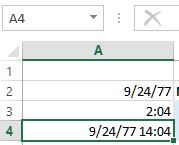The format of the date & time depends on the culture/zone you have selected while installing the operating system.

### How to get current date and time in Excel?

To get current date and time in MS Excel, we can use 'NOW()' function. Look at the example below. In B3 cell, we have written '=NOW()' that writes current date and time in Excel.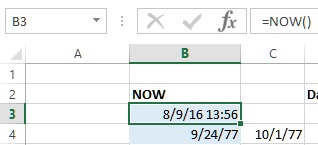### How to get Day, Month and Year from a Date in Excel?

Day, Month and Year can be extracted from a valid date.

DAY function

DAY function is used to return the day of month (1 to 31) from a valid date. D3 cell contains '=DAY(B3)' that returns '9' as day from B3 cell value.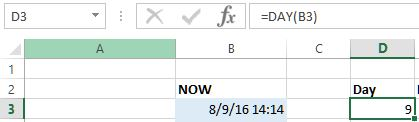MONTH function

MONTH function returns the month number from 1 (January) to 12 (December) from a validate date. E3 contains '=MONTH(B3)' formula that returns '8' as month from B3 cell value.YEAR Function

YEAR function is used to return year (1900 to 9999) from a valid date. F3 cell contains '=YEAR(B3)' formula that returns '2016' as year from B3 cell value.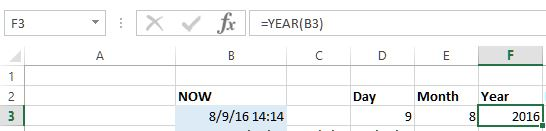### How to get Hour, Minute and Second from a Time in Excel?

HOUR function

HOUR function is used to return hour 0 (12:00 AM) to 23 (11:00 PM) from a valid time or date time. G3 cell contains '=HOUR(B3)' formula that returns '14' as hour from B3 cell value.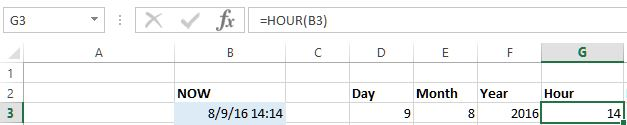MINUTE function

MINUTE function is used to return minute 0 to 59 from a valid time or date time. H3 cell contains '=MINUTE(B3)' formula that returns '16' as minute from B3 cell value.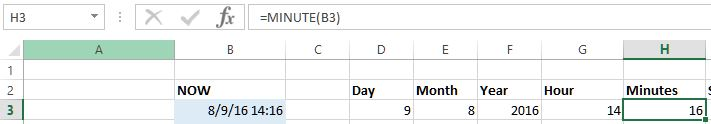SECOND function

SECOND function is used to return second 0 to 59 from a valid time or date time. I3 cell contains '=SECOND(B3)' formula that returns '25' as second from B3 cell value.

Even if B3 cell doesn't show second segment, Second is returned from the current time while writing the formula as B3 cell contains '=NOW()'.## Date and Time functions

DATE function

To add simply days into a date, write the formula as if you are trying to add a number into another number. Eg. C4 cell contains '=B4+7' that ia adding 7 days into '9/24/77' and giving the result.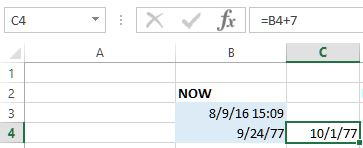To create a new date based on existing date segments, we can use DATE function. In D4 cell, we have written '=DATE(YEAR(B4)+5, MONTH(B4)+3, DAY(B4)+2)' formula that

1. adds 5 into B4 year
2. adds 3 into B4 month
3. adds 2 into B4 day
and gives a fresh date '12/26/82' based on '9/24/77'.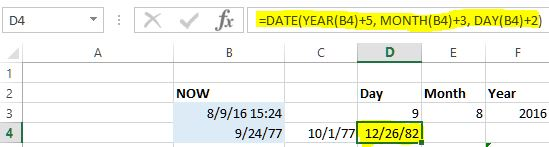like DATE, we have TIME function as well. TIME function can be used to get time based on other time values.

TIME function

Time function can be used to create a valid time based on integer numbers within time range. For example, G4 cell contains '=TIME(G3+2, H3+3, I3+3)' that

1. adds 2 into G3 value
2. adds 3 into H3 value
3. adds 3 into I3 value
and gives a fresh time '5:27 PM'.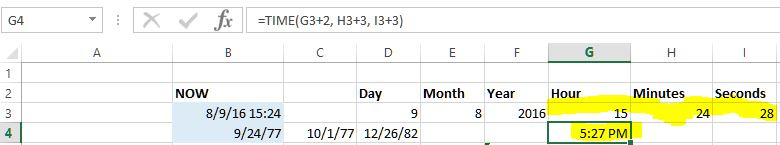### How to write different formats of date in Excel?

To write different date formats in Excel, we can use TEXT function. TEXT function accepts two parameters

• 1st - the value
• 2nd - the format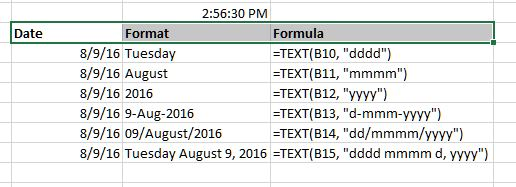When the value is a valid date and format is valid format,  TEXT function is intelligent enough to derive corresponding names of those date segments.

## Other DATE functions

1. TODAY() - returns today's date

2. EOMONTH() - returns the serial number of last day of the month, if 2nd parameter is not 0, it adds current month with that value and returns the last day of that month.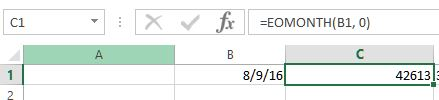You can see that C1 has serial number of the last day of the current month. To convert this serial number to date, I have used '=TEXT(C1, "dd-mmm-yyyy")' formula, and here is the result.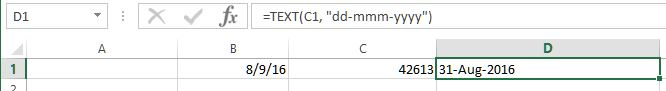To get next month last day, use '=EOMONTH(B1, 1)' that will return last day of September ie. 30-Sep-2016.

3. WEEKDAY() - returns the day of the week

4. DATEDIFF() - used to calculate the difference between two dates in days, months and years. In F2 cell, '=DATEDIF(\$B\$2, \$A\$2, "d")' formula is written that gets the difference between B2 and A2 cell (Notice that we have used Absolute reference while referencing the cell).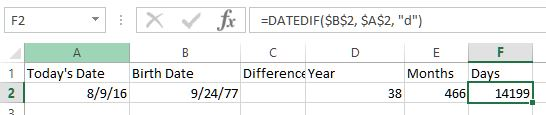Parameters are following
1st - lower date
2nd - higher date
3rd - "d" for day difference, "m" for month difference, "y" for year difference
Thanks for reading. Liked it? do share with your friends and colleagues!
Views: 5571 | Post Order: 22